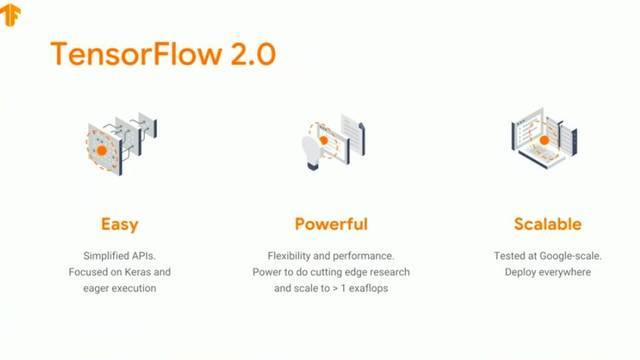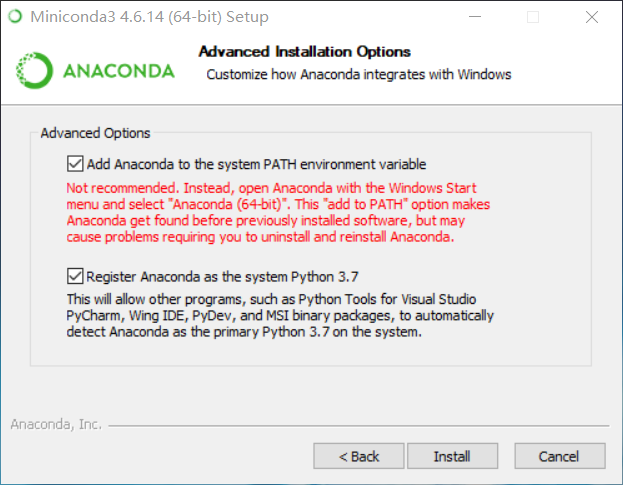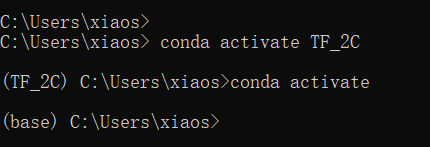# 『TensorFlow2.0正式版』极简安装TF2.0正式版（CPU&GPU）教程

## 0 前言

TensorFlow 2.0，今天凌晨，正式放出了2.0版本。## 1 环境准备

（关于ubuntu与mac版本的安装可以仿照此方法，因为conda支持多平台，应该没什么问题，如果大家问题多的话，可以评论，我后面会会更新ubuntu安装教程）

### 1.0 conda环境准备

conda是很好用python管理工具，可以方便建立管理多个python环境。后面安装的步骤里我也会介绍一些常用的conda指令。

conda 我推荐使用安装miniconda，大家可以理解为精简版的anaconda，只保留了一些必备的组件，所以安装会比快上很多，同时也能满足我们管理python环境的需求。（anaconda一般在固态硬盘安装需要占用几个G内存，花费1-2个小时，miniconda一般几百M，10分钟就可以安装完成了）

miniconda推荐使用清华源下载：https://mirrors.tuna.tsinghua.edu.cn/anaconda/miniconda/1. 查看conda环境：conda env list
2. 新建conda环境(env_name就是创建的环境名，可以自定义)：conda create -n env_name
3. 激活conda环境（ubuntu与Macos 将conda 替换为source）：conda activate env_name
4. 退出conda环境：conda deactivate
5. 安装和卸载python包：conda install numpy # conda uninstall numpy
6. 查看已安装python列表：conda list -n env_name

### 1.1 TF2.0 CPU版本安装

TF CPU安装比较简单，因为不需要配置GPU，所以windows ubuntu macOS安装方式都类似，缺点就是运行速度慢，但是用于日常学习使用还是可以的。

1.1.0 新建TF2.0 CPU环境（使用conda 新建环境指令 python==3.6表示在新建环境时同时python3.6）

conda create -n TF_2C python=3.6

1.1.1 进入TF_2C环境

conda activate TF_2C1.1.2 安装TF2.0 CPU版本（后面的 -i 表示从国内清华源下载，速度比默认源快很多）

pip install tensorflow==2.0.0 -i https://pypi.tuna.tsinghua.edu.cn/simple

1.1.3 测试TF2.0 CPU版本(把下面代码保存到demo.py使用TF_2C python运行)

import tensorflow as tf
version = tf.__version__
gpu_ok = tf.test.is_gpu_available()
print("tf version:",version,"\nuse GPU",gpu_ok)

tf version: 2.0.0
use GPU False

### 1.2 TF2.0 GPU版本安装

GPU版本和CPU类似，但是会多一步对于GPU支持的安装。下面来一步步实现。安装之前确认你的电脑拥有Nvidia的GPU

1.2.0 新建TF2.0 GPU环境（使用conda 新建环境指令 python==3.6表示在新建环境时同时python3.6）

conda create -n TF_2G python=3.6

1.1.1 进入TF_2G环境

conda activate TF_2G

1.1.2 安装GPU版本支持，拥有Nvidia的GPU的windows一般都有默认驱动的，只需要安装cudatoolkit 与 cudnn包就可以了，要注意一点需要安装cudatoolkit 10.0 版本，注意一点，如果系统的cudatoolkit小于10.0需要更新一下至10.0

conda install cudatoolkit=10.0 cudnn

1.1.3 安装TF2.0 GPU版本（后面的 -i 表示从国内清华源下载，速度比默认源快很多）

pip install tensorflow-gpu==2.0.0 -i https://pypi.tuna.tsinghua.edu.cn/simple

1.1.3 测试TF2.0 GPU版本(把下面代码保存到demo.py使用TF_2G python运行)

import tensorflow as tf
version = tf.__version__
gpu_ok = tf.test.is_gpu_available()
print("tf version:",version,"\nuse GPU",gpu_ok)

tf version: 2.0.0
use GPU True

### 1.2 最后我们测试一个使用TF2.0版本方式写的线性拟合代码

import tensorflow as tf

X = tf.constant([[1.0, 2.0, 3.0], [4.0, 5.0, 6.0]])
y = tf.constant([[10.0], [20.0]])

class Linear(tf.keras.Model):
def __init__(self):
super().__init__()
self.dense = tf.keras.layers.Dense(
units=1,
activation=None,
kernel_initializer=tf.zeros_initializer(),
bias_initializer=tf.zeros_initializer()
)

def call(self, input):
output = self.dense(input)
return output

# 以下代码结构与前节类似
model = Linear()
optimizer = tf.keras.optimizers.SGD(learning_rate=0.01)
for i in range(100):
y_pred = model(X)      # 调用模型 y_pred = model(X) 而不是显式写出 y_pred = a * X + b
loss = tf.reduce_mean(tf.square(y_pred - y))

if i % 10 == 0:
print(i, loss.numpy())
print(model.variables)

### 输出结果如下：

0 250.0
10 0.73648137
20 0.6172349
30 0.5172956
40 0.4335389
50 0.36334264
60 0.3045124
70 0.25520816
80 0.2138865
90 0.17925593
[<tf.Variable 'linear/dense/kernel:0' shape=(3, 1) dtype=float32, numpy=
array([[0.40784496],
[1.191065  ],
[1.9742855 ]], dtype=float32)>, <tf.Variable 'linear/dense/bias:0' shape=(1,) dtype=float32, numpy=array([0.78322077], dtype=float32)>]

## -1. 后记©️2019 CSDN 皮肤主题: 1024 设计师: 上身试试